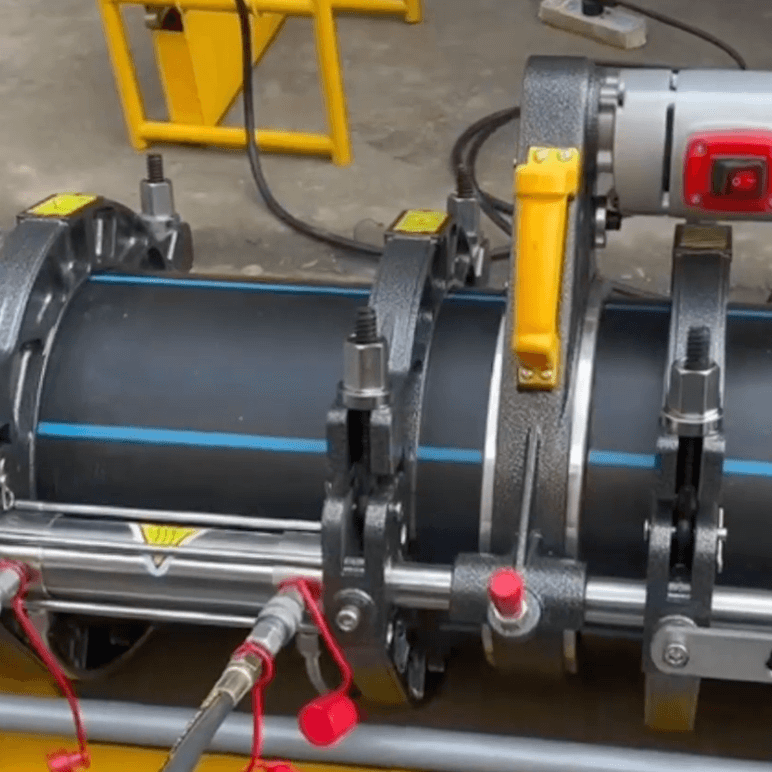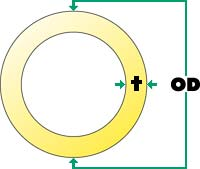## Butt Fusion Machine & Pipe Tool Manufacturer

Language:
Home / All / PRODUCT NEWS /

# Fusion Pressure Calculator

Jan 6,2023
Learn To Calculate Fusion Pressure
EXAMPLE
Using a 28 Machine from Chart A (at right) we will determine our gauge pressure.
Pipe Size = 8" IPS
OD of Pipe = 8.625
DR of Pipe = 11
IFP = 75 PSI
Measured Drag = 30 PSIVARIABLE DEFINITIONS
OD = Outside diameter
T = Wall thickness
PI = 3.1416
DR = Dimensional ratio
IFP = Manufacturers recommended interfacial pressure
TEPA = Total effective piston area
DRAG = Force required to move pipeTotal Effective Piston Area
Model High Force Standard Medium Force High Velocity Low Force Extra High Velocity
28 4.71 NA 1.67
412 11.78 6.01 3.14
618 11.78 6.01 3.14
500 NA 6.01 NA
824 29.44 15.32 9.45
1236 29.44 15.32 9.45
900 NA 15.32 NA
1648 31.42 14.14 NA
2065 31.42 NA NA
STEP 1. - CALCULATING WALL THICKNESS:
To determine wall thickness we will be using the Example (above).

T = OD/DR = Wall thickness
Now the Example and the formula above we will calculate the wall thickness.
T = 8.625/11 = .784

STEP 2. - FINDING TOTAL EFFECTIVE PISTON AREA
Use Chart A (above) to find the TEPA of your machine.

TEPA = 4.710 (we are using the 28 as an example)

STEP 3. - CALCULATING GAUGE PRESSURE
Using the information we have gathered from above.

{(OD - T) x T x PI x IFP}/TEPA        + DRAG = Gauge Pressure

STEP 4. - COMPLETED FORMULA
Now using all the information and the formula above we will find the gauge pressure.

{(8.625 - .784) x .784 x 3.1416 x 75}/4.710        + 30 PSI = 338 PSI
If you are working on a butt fusion project or have questions/issues relating to this process, contact our fusion specialists.
Language: